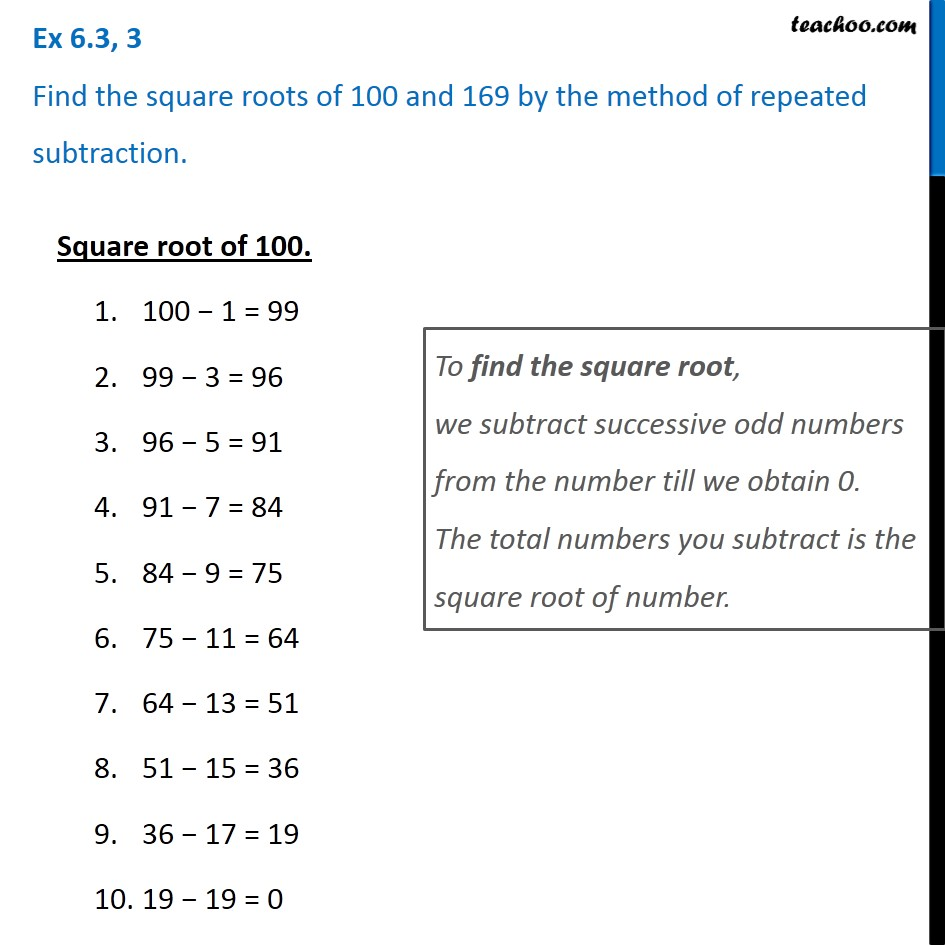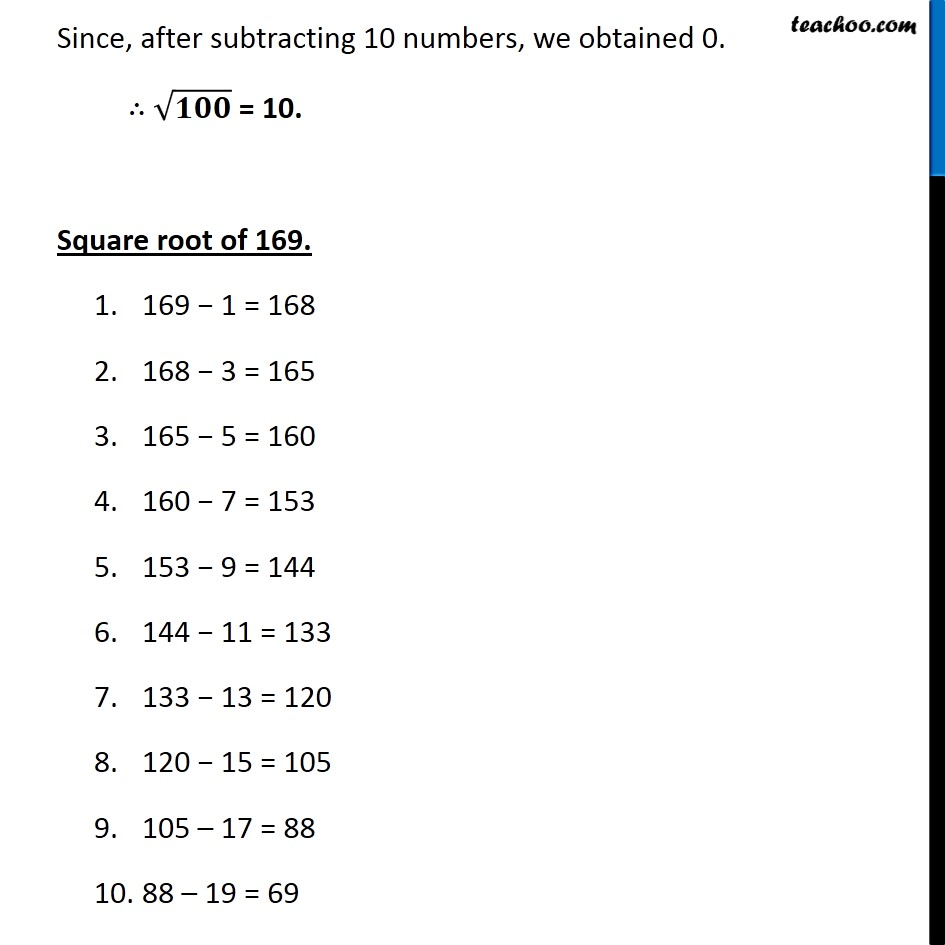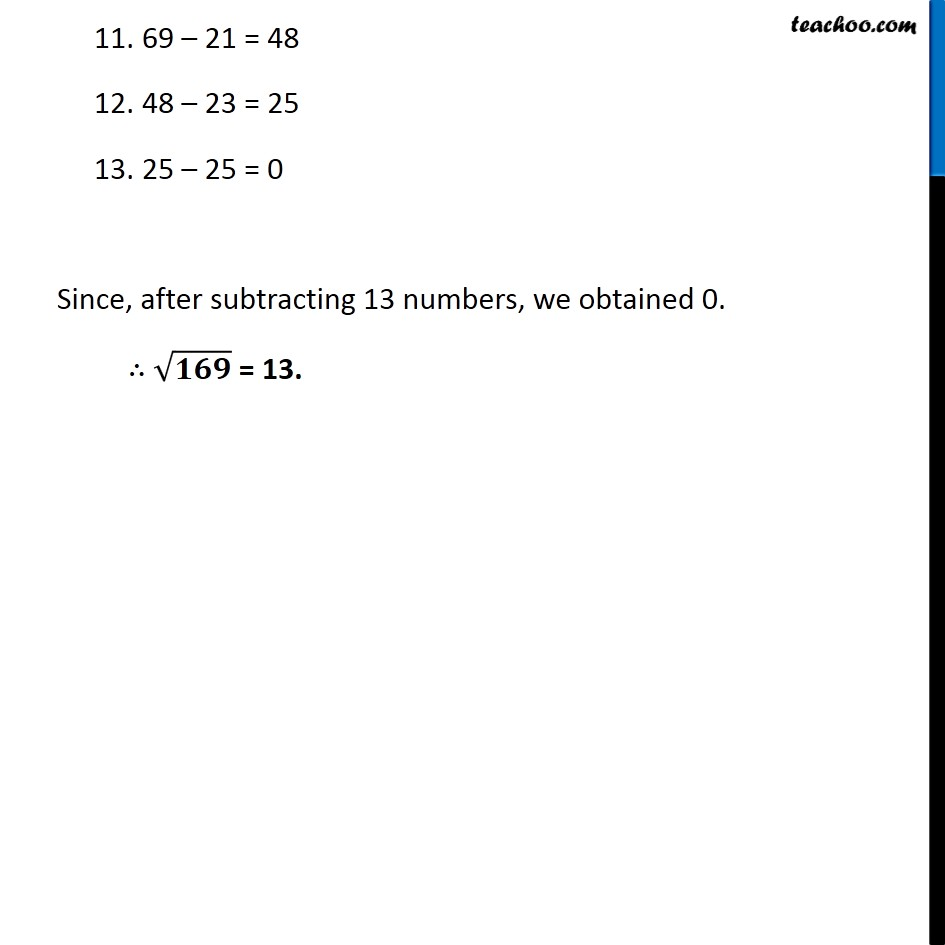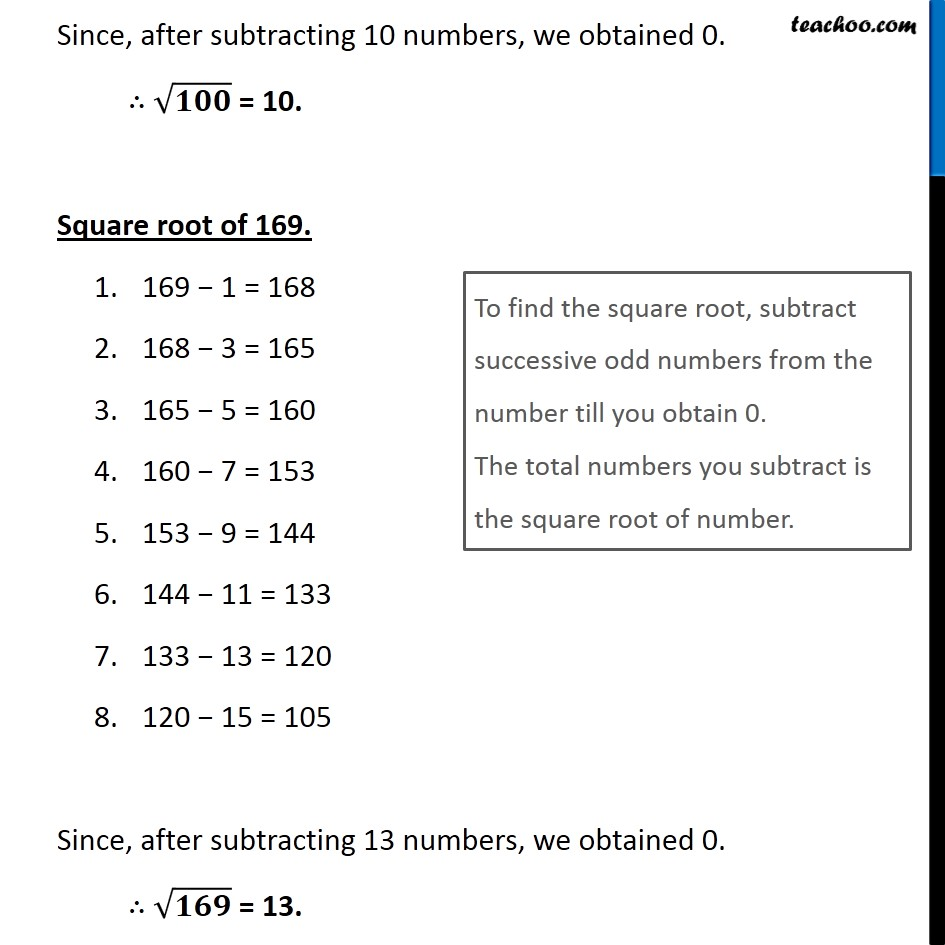Ex 5.3

Chapter 5 Class 8 Squares and Square Roots
Serial order wiseLearn in your speed, with individual attention - Teachoo Maths 1-on-1 Class

### Transcript

Ex 5.3, 3 Find the square roots of 100 and 169 by the method of repeated subtraction. Square root of 100. 100 − 1 = 99 99 − 3 = 96 96 − 5 = 91 91 − 7 = 84 84 − 9 = 75 75 − 11 = 64 64 − 13 = 51 51 − 15 = 36 36 − 17 = 19 19 − 19 = 0 To find the square root, we subtract successive odd numbers from the number till we obtain 0. The total numbers you subtract is the square root of number. Since, after subtracting 10 numbers, we obtained 0. ∴ √𝟏𝟎𝟎 = 10. Square root of 169. 169 − 1 = 168 168 − 3 = 165 165 − 5 = 160 160 − 7 = 153 153 − 9 = 144 144 − 11 = 133 133 − 13 = 120 120 − 15 = 105 105 – 17 = 88 88 – 19 = 69 69 – 21 = 48 48 – 23 = 25 25 – 25 = 0 Since, after subtracting 13 numbers, we obtained 0. ∴ √𝟏𝟔𝟗 = 13. Since, after subtracting 10 numbers, we obtained 0. ∴ √𝟏𝟎𝟎 = 10. Square root of 169. 169 − 1 = 168 168 − 3 = 165 165 − 5 = 160 160 − 7 = 153 153 − 9 = 144 144 − 11 = 133 133 − 13 = 120 120 − 15 = 105 Since, after subtracting 13 numbers, we obtained 0. ∴ √𝟏𝟔𝟗 = 13. Since, after subtracting 10 numbers, we obtained 0. ∴ √𝟏𝟎𝟎 = 10. Square root of 169. 169 − 1 = 168 168 − 3 = 165 165 − 5 = 160 160 − 7 = 153 153 − 9 = 144 144 − 11 = 133 133 − 13 = 120 120 − 15 = 105 Since, after subtracting 13 numbers, we obtained 0. ∴ √𝟏𝟔𝟗 = 13. To find the square root, subtract successive odd numbers from the number till you obtain 0. The total numbers you subtract is the square root of number.